ANGSD: Analysis of next generation Sequencing Data

Latest tar.gz version is (0.938/0.939 on github), see Change_log for changes, and download it here.

# ErrorSykMethod

The likelihood of the sequencing data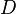$D$ of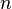$n$ individuals for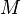$M$ sites can be described through the allele frequencies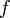$f$ and the type specific error rates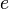$e$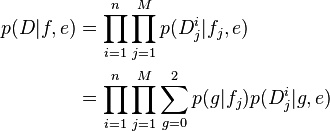\begin{align} p(D|f,e) &= \prod_{i=1}^n \prod_{j=1}^M p(D_j^i|f_j,e)\\ &= \prod_{i=1}^n \prod_{j=1}^M \sum_{g=0}^2 p(g|f_j)p(D_j^i|g,e) \end{align}
by summing over the unknown genotypes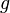$g$. The genotype likelihood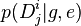$p(D_j^i|g,e)$ relies on the type specific error rates (see kim2011 p.14 for details). The type specific error rates are obtain along site the allele frequencies by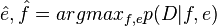$\hat{e},\hat{f} = argmax_{f,e} p(D|f,e)$